# Checking if Array is Sorted in C

## Checking if Array is Sorted in C Language:

In this article, I am going to discuss Checking if Array is Sorted in C Language with Examples. In our previous article, we have seen the Reverse, Shifting, and Rotation in an Array with Examples. In this article, we will also see some operations which we can perform on a sorted array i.e.

1. Inserting in a sorted array.
2. Checking if an array is sorted.
3. Arranging –ve on the left side in an array.

So, these are a few simple operations but they are important operations. So, let’s discuss these operations one by one.

##### 1. Insert Operations in a Sorted Array–

We want to insert a new value in an array that is already sorted. So, we want to add a new value at a sorted position. We want to insert a new element 18 in the below-given array: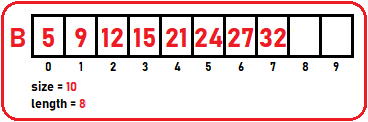The above array is already sorted. So, we have to insert 18 in the above array such that it will insert at the sorted position. So, we will compare 18 to each element in the array and check where it should be inserted. 18 is less than 21 and greater than 15 so it should insert before 21 and after 15. But there is no space for insertion. Now, we will shift all the elements after 15 towards the right side so that 18 can be inserted at the sorted position.

For shifting, we should start from the last element. We can simply check for this number from the last element onwards. If those elements are greater than this one, we can shift them. Suppose this is not 18, this is 38. So, this is greater than 32 so we can simply store 38 at the last position. But 18 is smaller so we can start shifting: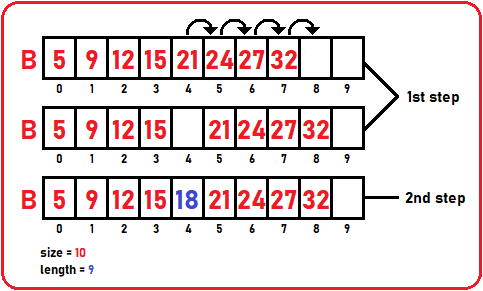We check for until and unless 18 will be greater than the current element. If 18 is greater then we will stop there and insert 18 at the next position.

Note: we will not check from the left-hand side because if we do so then also, we have to shift right-hand side elements so better to start comparing from the right side of the array. Now, let’s discuss the procedure for insertion: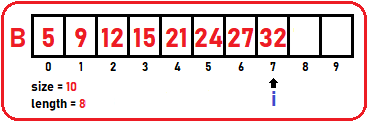First, we will initialize i to the last position of the array and check for if (B [i] > 18) then shift the element. Now, we will decrement i: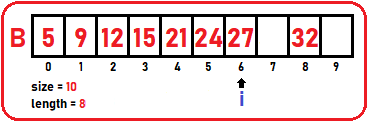In the same manner, i will decrement to the element which will be lower than 18: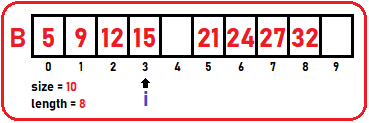Now, from the above image, we want to insert 18 at 4th or i + 1 so increment i for only one time after the loop ends: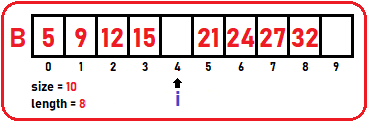Now i is pointing to the correct index, here we will insert our element which is 18: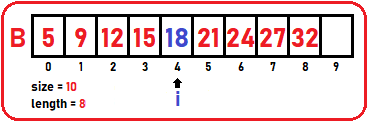##### Insert Operation Code in a Sorted Array in C Language:
```void InsertSort(struct List *list, int x){
int i = list->length-1;

if(list->length == list->size)
return;
while(i >= 0 && list->B[i] > x)	{
list->B[i+1] = list->B[i];
i--;
}
list->B[i+1] = x;
list->length++;
}```
##### 2. Checking if an array is sorted –

For checking the array or list is sorted or not, start checking from 1st element. Below is an array of size 10: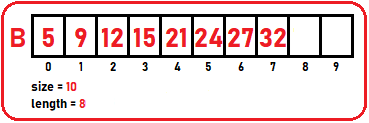In the above array, the first element is smaller than the next element and the next element is smaller than the next element i.e. B [i] < B [i+1]. Every current element is smaller than the next element. Then it means the list or array is sorted. If we modify the 5th element to 26: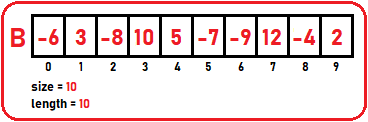Then here list will not be a sorted list because the 5th element is not smaller than the 6th element. We have to stop one element before the last element i.e. n-2

In our program, we will check for false condition i.e. if (B [i] > B [i+1]). If we never find any element that is greater than its next element then the array will be a sorted array otherwise not.

##### isSorted Code:
```int isSorted(struct List list){
int i;
for(i=0;i<list.length-1;i++){
if(list.B[i]>list.B[i+1])
return 0;
}
return 1;
}
```

Time Complexity: O (n)

##### 3. Arranging –ve numbers on the left side in an array –

We will arrange all negative elements on the left-hand side and positive elements on the right side.As we can see, both –ve and +ve numbers are randomly positioned. So, we have to arrange them accordingly. To arrange them, we can take two pointers that are index pointers. One is i at beginning of the list and the second is j at the end of the list: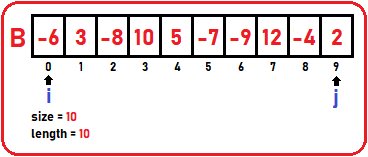Using i we are looking for +ve numbers, if there are any +ve numbers on the left side, let us send them on the right side. And with j we will find out if there are any –ve numbers on the right side, so let us send them on the left side. i will try to find a positive number and j will try to find the negative number and if found then they will exchange. Let see the procedure:is i pointing to +ve number? No, increment i: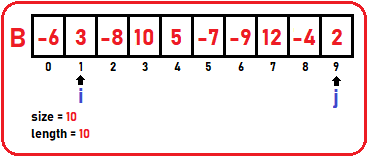is i pointing to +ve number? Yes. Is j pointing to –ve number? No, decrement j: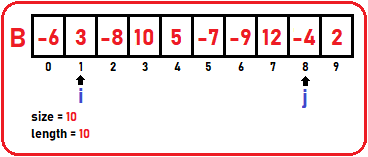Is j pointing to –ve number? Yes. Now j pointing to –ve number and i pointing to +ve number so interchange their elements: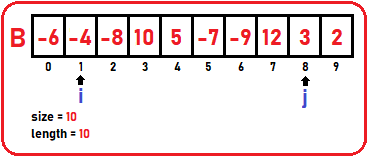In the same manner, all the +ve and –ve numbers will interchange and then arrange according to our condition.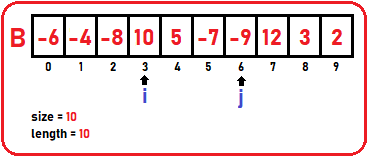Here, i is pointing to +ve number and j is pointing to –ve number, so we have to interchange them: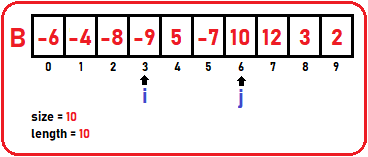At last, we will get our array in which –ve numbers are arranged on left side and +ve numbers are arranged on right side: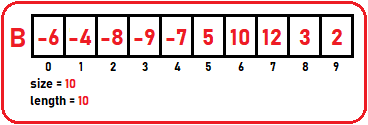##### Arranging –ve numbers on the left side in an array Code:
```void arrangeNegPos(struct List *list){
int i, j;
i = 0;
j = list->length - 1;
while(i < j){
while (list->B[i] < 0) i++;
while (list->B[i] >= 0) j--;
if (i < j) swap (&list->B[i], &list->B[j]);
}
}
```

Time Complexity: O (n)

##### Full Code in C language:
```#include<stdio.h>
#include<stdlib.h>

struct List{
int B;
int size;
int length;
};

void Display(struct List list){
int i;
printf("\nElements are\n");
for(i=0;i<list.length;i++)
printf("%d ",list.B[i]);
}

void swap(int *x,int *y){
int temp=*x;
*x=*y;
*y=temp;

}

void InsertSort(struct List *list, int x){
int i = list->length-1;

if(list->length == list->size)
return;
while(i >= 0 && list->B[i] > x)	{
list->B[i+1] = list->B[i];
i--;
}
list->B[i+1] = x;
list->length++;
}

int isSorted(struct List list){
int i;
for(i=0;i<list.length-1;i++){
if(list.B[i]>list.B[i+1])
return 0;
}
return 1;
}

void arrangeNegPos(struct List *list){
int i, j;
i = 0;
j = list->length - 1;
while(i < j){
while (list->B[i] < 0) i++;
while (list->B[j] >= 0) j--;
if (i < j) swap (&list->B[i], &list->B[j]);
}
}

int main()
{
struct List list1={{2,3,9,16,18,21,28,32,35},10,9};

printf("Before Insert:");
Display(list1);

printf("\n\n");

InsertSort(&list1, 25);

printf("After Insert:");
Display(list1);

return 0;
}
```

In the next article, I am going to discuss Merging Arrays in C Language with Examples. Here, in this article, I try to explain Checking if Array is Sorted in C Language with Examples as well as we also discussed Sorted Array Operations and I hope you enjoy this Checking if Array is Sorted in C Language with Examples article.